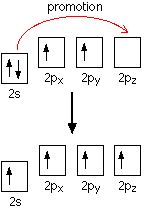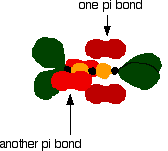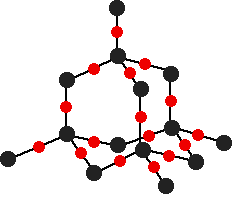# Oxides of Group 4 Elements

•• Contributed by Jim Clark
• Former Head of Chemistry and Head of Science at Truro School in Cornwall

This page briefly examines the oxides of carbon, silicon, germanium, tin and lead. It concentrates on the structural differences between carbon dioxide and silicon dioxide, and on the trends in acid-base behavior of the oxides down Group 4.

## The structures of carbon dioxide and silicon dioxide

The physical properties of carbon dioxide differ significantly from those of silicon dioxide (also known as silicon(IV) oxide or silica). Carbon dioxide is a gas whereas silicon dioxide is a hard, high-melting solid. The other dioxides in Group 4 are also solids, making the structure of carbon dioxide the anomaly.

### The structure of carbon dioxide

The fact that carbon dioxide is a gas indicates that it consists of small, simple molecules. Carbon can form these molecules because it can form double bonds with oxygen.None of the other elements in Group 4 form double bonds with oxygen, so their oxides adopt completely different structures. When carbon forms bonds with oxygen, it promotes one of its 2s electrons into the empty 2p level. This produces 4 unpaired electrons.These electrons are rearranged by hybridizing the 2s electron and one of the 2p electrons to make two sp1 hybrid orbitals of equal energy. The other 2p electrons are unaffected during this process.The figure below illustrates this:Notice that the two green lobes are two different hybrid orbitals, arranged as far from each other as possible (although the two hybrid orbitals have an arrangement similar to a p orbital, it is important not to confuse the two).

Oxygen's electronic structure is 1s22s22px22py12pz1, and its orbitals must also hybridize. In this case, sp2 hybrids are formed from the s orbital and two of the p orbitals, rearranging to form 3 orbitals of equal energy, leaving a temporarily unaffected p orbital.As shown below, two of the sp2 hybrid orbitals contain lone pairs of electrons.In the figure below, the carbon and oxygen atoms are arranged in pre-bonding position:The green hybrid orbitals overlap end-to-end, forming covalent bonds. These are called sigma bonds, and are shown as orange in the next diagram. The sigma bonding brings the p orbitals close enough to overlap.This overlap between the two sets of p orbitals produces two $$pi$$ bonds, similar to the $$pi$$ bond found in ethene. These $$pi$$ bonds are twisted at 90° to each other in the final molecule.To form a carbon-oxygen double bond, it is necessary for the lobes of the p orbitals on the carbon and the oxygen to overlap correctly.

## The structure of silicon dioxide

Silicon does not double bond with oxygen. Because silicon atoms are larger than carbon atoms, silicon-oxygen bonds are longer than carbon-oxygen bonds. Consider a hypothetical silicon-oxygen double bond, analogous to the carbon-oxygen double bond discussed above. Because silicon-oxygen bonds are longer than carbon-oxygen equivalents, the p orbitals on silicon and oxygen cannot overlap enough to form a stable pi bond. Therefore, only single bonds are formed.

There are several structures for silicon dioxide. One of the simplest is shown below:This is similar to the structure of diamond, with each of the silicon atoms bridged to its four neighbors via an oxygen atom, forming a large network covalent structure. Strong bonds in three dimensions make silicon dioxide a hard, high melting point solid.

## The acid-base behavior of the Group 4 oxides

The oxides of the elements at the top of Group 4 are acidic, but this acidity decreases down the group. Toward the bottom of the group the oxides are more basic, but do not lose their acidic character completely. A compound with both acidic and basic properties is called amphoteric. The trend, therefore, ranges from acidic oxides at the top of the group toward amphoteric oxides at the bottom.

## Carbon and silicon oxides

### Carbon monoxide

Carbon monoxide is usually treated as a neutral oxide, but it is slightly acidic. It does not react with water, but it can react with hot concentrated sodium hydroxide solution to give a solution of sodium methanoate.

$NaOH + CO \rightarrow HCOONa$

The reaction of carbon monoxide with basic hydroxide ions displays its acidic character.

### Dioxides of Carbon and Silicon

These compounds are both weakly acidic.

#### With water

• Silicon dioxide does not react with water, due to the energetic difficulty of breaking up the giant covalent structure.
• Carbon dioxide reacts slightly with water to produce hydrogen ions (strictly hydronium ions) and bicarbonate ions.

The chemical equation for CO2 is given below:

$H_2O (l) + CO_2(aq) \rightleftharpoons H^+(aq) + HCO_3^- (aq)$

The solution of carbon dioxide in water is sometimes known as carbonic acid, but in fact only about 0.1% of the carbon dioxide actually reacts. The equilibrium lies well to the left.

#### With bases

At low temperatures, carbon dioxide reacts with sodium hydroxide to form solutions of either sodium carbonate or sodium bicarbonate, depending on the proportions used. Equations for these reactions are given below:

$2NaOH + CO_2 \rightarrow Na_2CO_3 + H_2O$

$NaOH + CO_2 \rightarrow NaHCO_3$

At high temperatures and concentrations, silicon dioxide also reacts with sodium hydroxide, forming sodium silicate as shown:

$2NaOH + SiO_2 \rightarrow Na_2SiO_3 + H_2O$

Another familiar reaction occurs in the Blast Furnace extraction of iron; in this process, calcium oxide (from limestone, one of the raw materials) reacts with silicon dioxide to produce a liquid slag of calcium silicate. This is another example of acidic silicon dioxide reacting with a base.

$CaO(s) + SiO_2(s) \rightarrow CaSiO_3 (l)$

## Germanium, tin and lead oxides

### The monoxides

These elements form amphoteric oxides.

#### The basic nature of the oxides

These oxides react with acids to form salts. An example of this reaction, using hydrochloric acid, is given below:

$XO(s) + 2HCl(aq) \rightarrow XCl_2(aq) + H_2O (l)$

where \ (X\) represents Ge or Sn; a modification is required for lead.

When lead(II) oxide is mixed with hydrochloric acid, an insoluble layer of lead(II) chloride forms over the oxide; this stops the reaction from proceeding. A more accurate chemical equation from that given above is the following:

$PbO(s) + 2HCl(aq) \rightarrow PbCl_2 (s) + H_2O$

However, if a high enough concentration of HCl is used, the large excess of chloride ions can react with lead(II) chloride to produce soluble complexes such as PbCl42-. These ionic complexes are soluble in water, so the reaction continues. The equation for this process is given below:

$PbCl_2 (s) + 2Cl^-(aq) \rightarrow PbCl_4^{2-} (aq)$

#### The acidic nature of the oxides

These oxides also react with bases like sodium hydroxide, without exception, as follows:

$XO(s) + 2OH^-(aq) \rightarrow XO_2^{2-}(aq) + H_2O(l)$

Lead(II) oxide, for example, reacts to form PbO22- (plumbate(II)) ions.

### The dioxides

These dioxides are amphoteric, as mentioned before.

#### The basic nature of the dioxides

These dioxides react first with concentrated hydrochloric acid to form compounds of the type XCl4:

$XO_2 + 4HCl \rightarrow XCl_4 + 2H_2O$

These XCl4 compounds react with excess chloride ions in the hydrochloric acid to form complexes such as XCl62-.

$XCl_4 + 2Cl^- \rightarrow XCl_6^{2-}$

In the case of lead(IV) oxide, the reaction requires ice-cold hydrochloric acid. If the reaction is carried out at warmer temperatures, the lead(IV) chloride decomposes to give lead(II) chloride and chlorine gas. This is because the preferred oxidation state of lead is +2 rather than +4.

#### The acidic nature of the dioxides

The dioxides react with hot concentrated sodium hydroxide, forming soluble complexes of the form [X(OH)6]2-.

$XO_2(s) + 2OH^-(aq) + 2H_2O (l) \rightarrow [X(OH)_6]^{2-} (aq)$

Some sources suggest that lead(IV) oxide requires molten sodium hydroxide to react. In that case, the equation is modified as follows:

$PbO_s (s) + 2NaOH (l) \rightarrow Na_2PbO_3(s) + H_2O (g)$